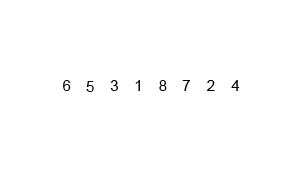# Recursive merge sort implementation in c#

In this article, I will show you the implementation of `Merge Sort` the algorithm in C#.

## What is merge sort?

Merge sort is a divide-and-conquer algorithm based on the idea of breaking down a list into several sub-lists until each sublist consists of a single element and merging those sublists in a manner that results into a sorted list.

## Merge sort Animation``````using System;
using System.Collections.Generic;
using System.Linq;
using System.Text;

namespace MergeSort
{
public class Program
{
private int[] originalArray;
private int[] resultArray;

public void MergeSort(int[] array)
{
this.originalArray = array;
this.resultArray = new int[array.Length];
Merge(0, array.Length - 1);
}

private void Merge(int low, int high)
{
if (low < high)
{
int middle = (low + high) / 2;
Merge(low, middle);
Merge(middle + 1, high);
Sort(low, middle, high);
}
}
/// <summary>
/// Returns a new sorted list containing the same elements as L
/// </summary>
/// <param name="low"></param>
/// <param name="middle"></param>
/// <param name="high"></param>
private void Sort(int low, int middle, int high)
{

for (int index = low; index <= high; index++)
{
resultArray[index] = originalArray[index];
}
int i = low;
int j = middle + 1;
int k = low;
while (i <= middle && j <= high)
{
if (resultArray[i] <= resultArray[j])
{
originalArray[k] = resultArray[i];
i++;
}
else
{
originalArray[k] = resultArray[j];
j++;
}
k++;
}
while (i <= middle)
{
originalArray[k] = resultArray[i];
k++;
i++;
}
}
public static void Main(String[] args)
{
int[] arr = { -4, 7, -9, 11, 8, 22, 98, 5 };

Program example = new Program();
example.MergeSort(arr);
}
}

}
``````